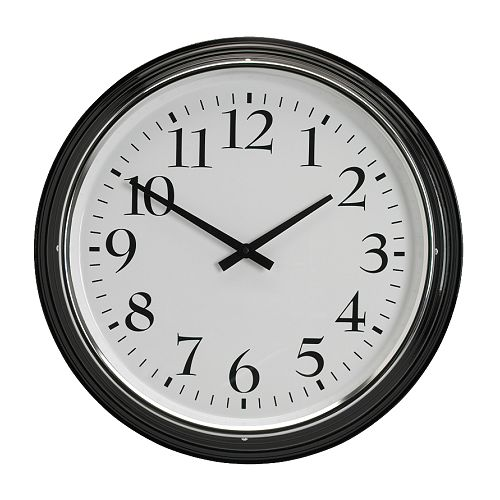# Third Grade Math Pre-test Quiz

7 Questions | Total Attempts: 2403SettingsThis test will be used to see how much you remember from 2nd grade and need to review in 3rd grade!

• 1.
What is the place value of 7 in the following number: 7,345
• A.

Ones

• B.

Hundreds

• C.

Thousands

• D.

Tens

• 2.
Which number would complete this pattern? 240, 245,____, 255. 260, 265
• A.

246

• B.

250

• C.

200

• D.

270

• 3.
Molly scored 50 points in a game. Her sister scored double that number of points. How many points did her sister score score?
• A.

50

• B.

100

• C.

150

• D.

200

• 4.
What time does this clock show?
• A.

10:10

• B.

2:10

• C.

5:50

• D.

1:50

• 5.
Which figure has a line of symmetry?
• A.

A

• B.

B

• C.

C

• D.

D

• 6.
(7+4)+3 = (3+7) +4
• A.

True

• B.

False

• 7.
What is the sum of the following problem:   35 +78
• A.

102

• B.

158

• C.

113

• D.

103

Related TopicsBack to top